Transcripted Summary

We've seen one basic control structure in JavaScript, which is the `for` loop. What we're going to look at now is the `if` statement.

If we have a `for` loop, which can loop through a whole bunch of items; and we have an `if` statement which can make decisions on them, we probably got all the control flows that we actually need in order to make some sensible automated execution for this application.

Let's have a quick look — I've cleared the data down to get this nice and simple again.

So, an `if` statement, I'll just show you in the console — `if`, open brackets the actual condition we want to put in the `if` statement [`if(true)`]. So, this would be any true statement, then (there's no then keyword is just in brackets) and then I'll say `console.log`, “hello” — `if(true){console.log(“hello”)}`

If I want to format this, let me put the new lines in, there we go. I'm going to put in a semicolon, don't need to, but I tend to ... some spaces. Format your code nicely.

``````if(true){
console.log("hello");
}
``````

Now if you're working in console, you typically don't format your code, but I'm formatting the code to make it readable for you.

So true evaluates to true.

``````if(false){
console.log("hello");
}
``````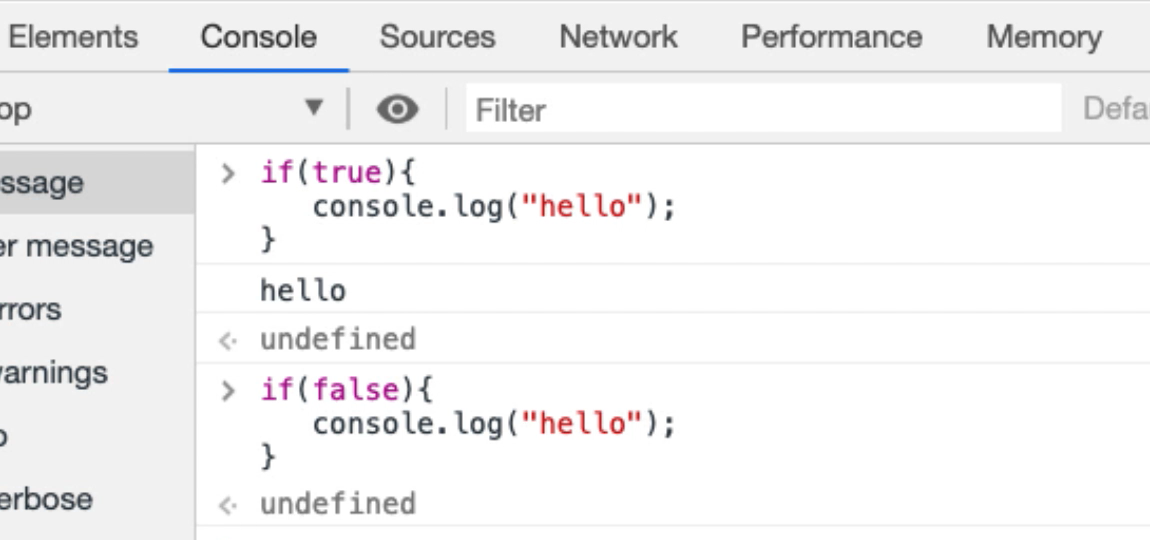False evaluates to false, so nothing got printed out.

I want to be able to do conditions, so I want to see things like, “if, something, one equals one, then hello.”

``````if(1===1){
console.log("hello");
}
``````

Now JavaScript is a little bit fussy about its equal signs and you can put in 3.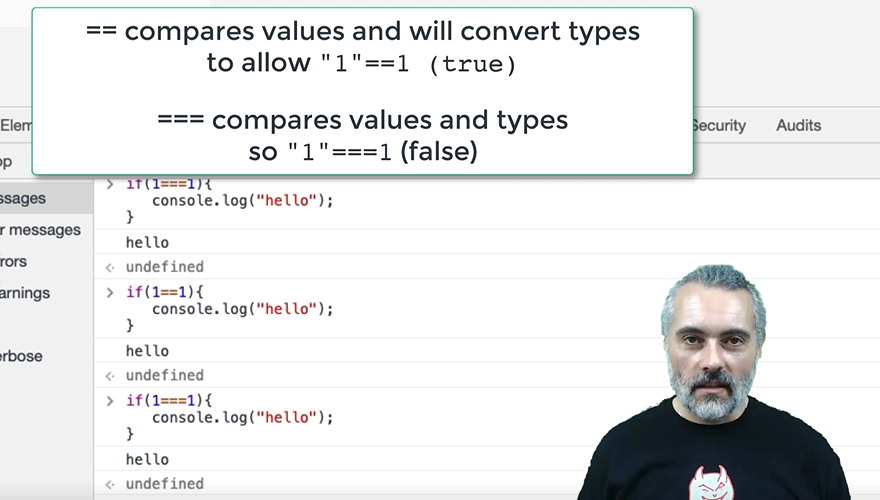So, if you’ve got:

``````if(1==1){
console.log("hello");
}
``````

that would try to set 1 to 1, we don't want to do that.

If, “1 equals 1”, that should evaluate to true.

``````if(1==1){
console.log("hello");
}
``````

If, one really equals one, then “hello”.

``````if(1===1){
console.log("hello");
}
``````

In JavaScript you'll have to look up the difference between double equals and triple equals and because JavaScript notion of truth and true.

Let’s use a variable: `var doit=true`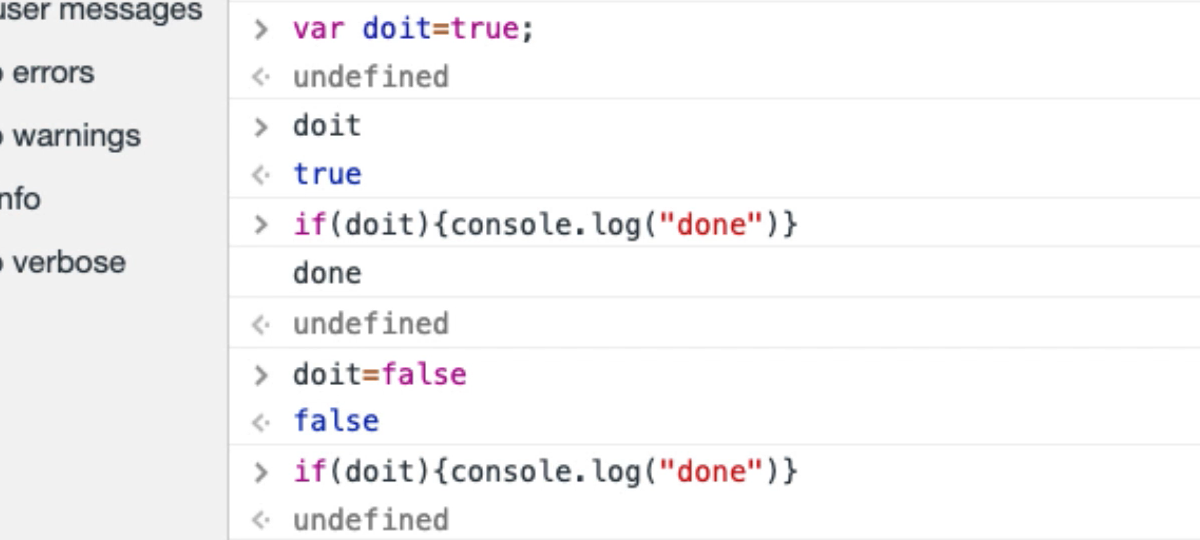What is `doit`? `doit` equals true.

So, I'm going to say:

``````var doit=true;
if(doit){
console.log("done");
}
``````

So, “doit” is true, so we've “done” it.

Let's set do it to equal to false.

``````var doit=false;
if(doit){
console.log("done");
}
``````

Is do it true? No, it isn't.

Okay, so that's pretty much an `if` statement.

And sometimes I'll use double equals, sometimes I'll use triple equals, if it works with double equals, I pretty much just leave it.

Remember, I'm not a proper JavaScript programmer, but the reason I'm pointing that out to you is I have managed to automate applications in the browser without really knowing a lot of JavaScript .

Let's take the `for` loop construct that we had before, and what I'm going to do is I'm going to toggle every second item in the loop.

I need some items here, so I'm going to say “todo”, “todo also”, “todo as well” and “todo yes”.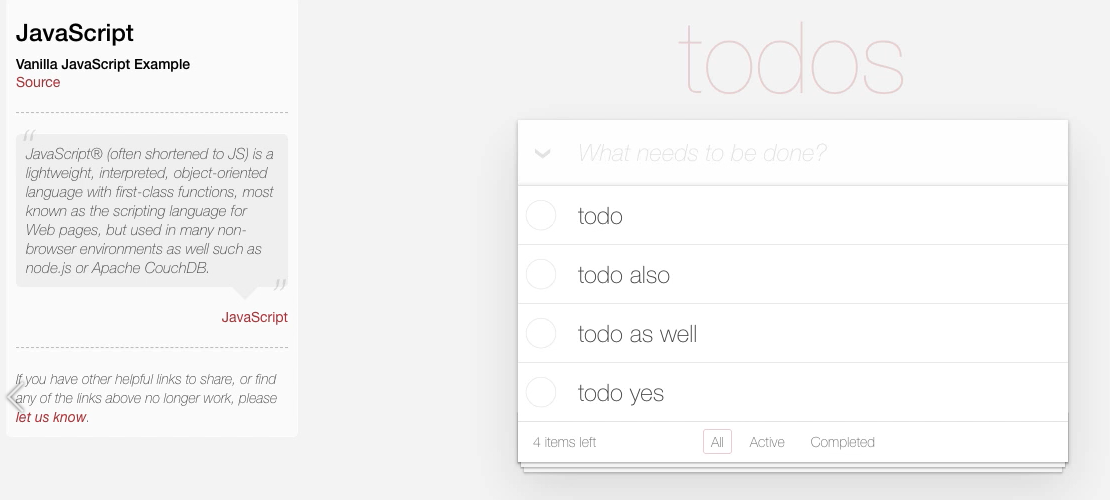I want to iterate over this list using a `for` loop and choose every second item.

### # Modulus Command

Now I'm going to do that by using a modulus command [`%`].

This was a common command that we used to use when we were writing games, programming in the olden days, in order to make sure things didn't go off the screen or to rotate sprites.

I'm just going to show you what that means just in case you don't know.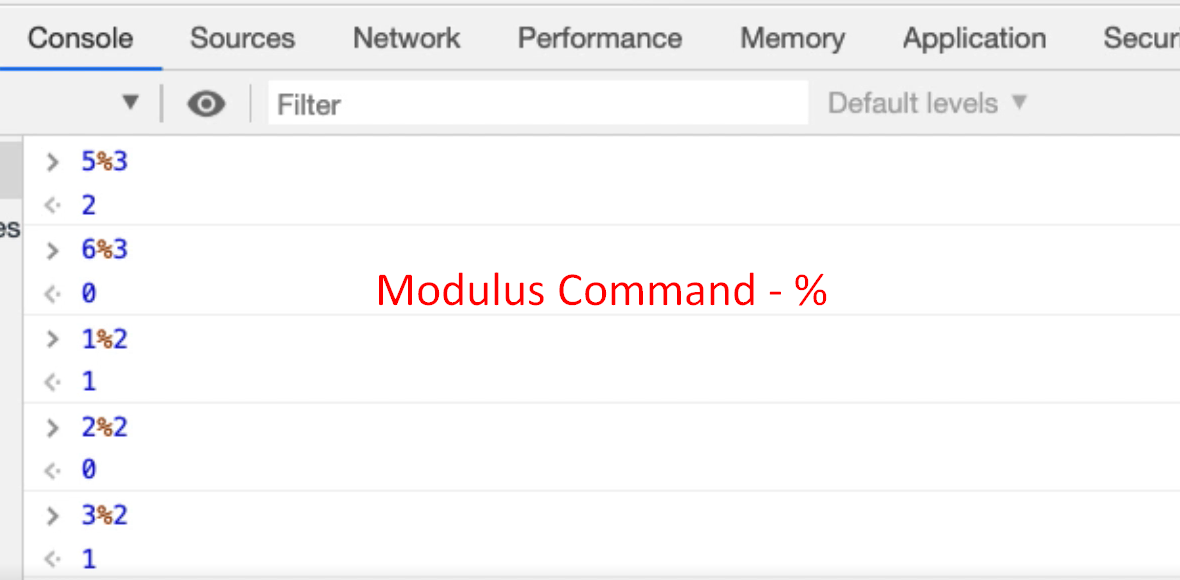Five modulus three — `5%3` — it's not 5 percent 3, it's 5 percent sign 3.

It’s basically saying 5, divided by 3, but give me the integer remainder. So, 3 goes into 5 once, with a remainder 2.

Six modulus three — `6%3` —3 goes into 6, 2 remainder 0.

Now if I do modulus two [%2], I can find out whether a number is odd or even.

• One modulus two — `1%2`— is 1; 2 doesn't go in, remainder 1.
• Two modulus two — `2%2` — 2 goes into 2 once, remainder 0.
• Three modulus two — `3%2` — 2 goes into 3 once, remainder 1.

Now I can tell whether an integer is odd or even.

I can use that to go through here — if it's an odd number, don't click it; if it's an even number, do.

So, I have in my scratch pad made a note of the `for` loop that toggles every item and what I want to do is say if it's an even number, then I will click it.

``````var toggles = document.querySelectorAll('.toggle');
for(togglepos=0;togglepos<toggles.length;togglepos++){
if(togglepos%2==0){
toggles[togglepos].click();
}
}
``````

I made a syntax error, so if I run that now, copy and paste that into my console. I made a mistake [it selects the odd]

Because it's zero indexed, the first item is 0.

I want to say if it's a 1 — so 0 is an even number, 1 is odd — I want to click that.

Let's set this back to normal.

``````// select every second item in the list
var toggles = document.querySelectorAll('.toggle');
for(togglepos=0;togglepos<toggles.length;togglepos++){
if(togglepos%2==1){
toggles[togglepos].click();
}
}
``````

There we go.

Now I make these mistakes as deliberate mistakes so that you can spot them in advance.

I could also have done that by incrementing this in a different way — I don't necessarily need an if statement to do that.

I could have said start at 0. Instead of just adding 1, which we'd increment on each one, I'm going to add 2.

``````var toggles = document.querySelectorAll(".toggle");
for(togglepos=0; togglepos<toggles.length; togglepos+=2){
toggles[togglepos].click();
}
``````

That's what adding 2 onto something looks like.

Let's try this.

We're saying click this one, don't click this one. Click this one, don't click this one.

So, this isn't every second one, this is everyone — to to get every second one, I need to start on the second one.

``````var toggles = document.querySelectorAll(".toggle");
for(togglepos=1; togglepos<toggles.length; togglepos+=2){
toggles[togglepos].click();
}
``````

So, if I start on 1 and add 2.

There you go. So now it's doing every second one.

I could have done it with a way that might seem a little bit more readable.

``````var toggles = document.querySelectorAll(".toggle");
for(togglepos=1; togglepos<toggles.length; togglepos= togglepos+2){
toggles[togglepos].click();
}
``````

That works fine.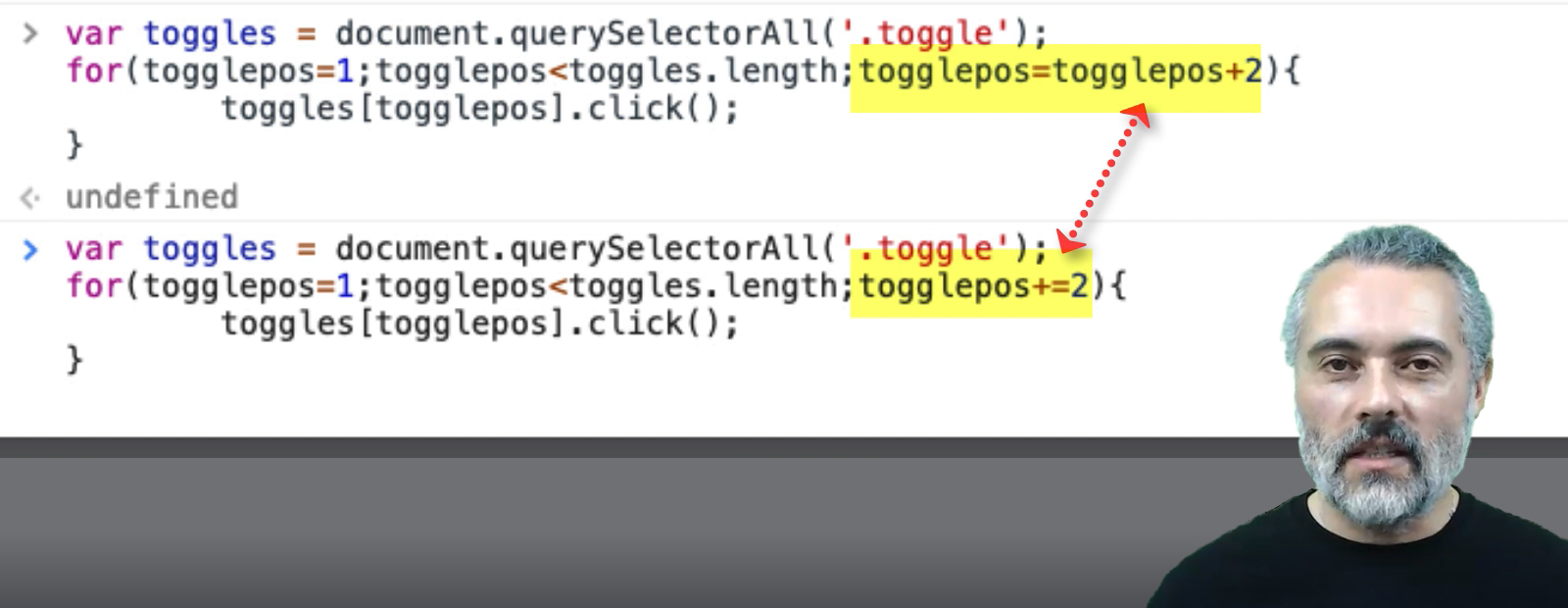It's just that the “plus equals two” [`+=2`] is a shorthand that you eventually learn how to write and rely on.

I could have done it with a temporary variable.

Let's get all the code back.

I could have said, let me write this in here because it's a little bit hard to write long lines in the console.

I'm going to say `var` “toggle it”, then I'll say the first one I didn't want to toggle. Do not want to toggle the first one. So, if we want to toggle it, then toggle it.

Then I want to say toggle it equals the reverse of what it did. So, equals not toggle it.

``````var toggles = document.querySelectorAll(".toggle");
var toggleit = false;
for(togglepos=0; togglepos<toggles.length; togglepos++){
if(toggleit){
toggles[togglepos].click();
}
toggleit=!toggleit;
}
``````

So, what happens is we say I didn't want to toggle this one. Okay, come through then reverse it. Next time it comes through and says I do want to toggle it, then I click on it.

If I run this, it should be good.

Here we go.

• We used a modulus statement to determine whether it's an odd or an even number, in order to toggle it.

• I incremented the list by 2 instead of 1 in order to skip over items

• I used a temporary variable — so you've seen an example of an `if` statement there.

And that's good enough `if` statement in order to let us do quite a lot of work in here.

If I ever want to do an `else` statement, I can say:

``````if(true){
console.log("true it is");
} else {
console.log("false indeed");
}
``````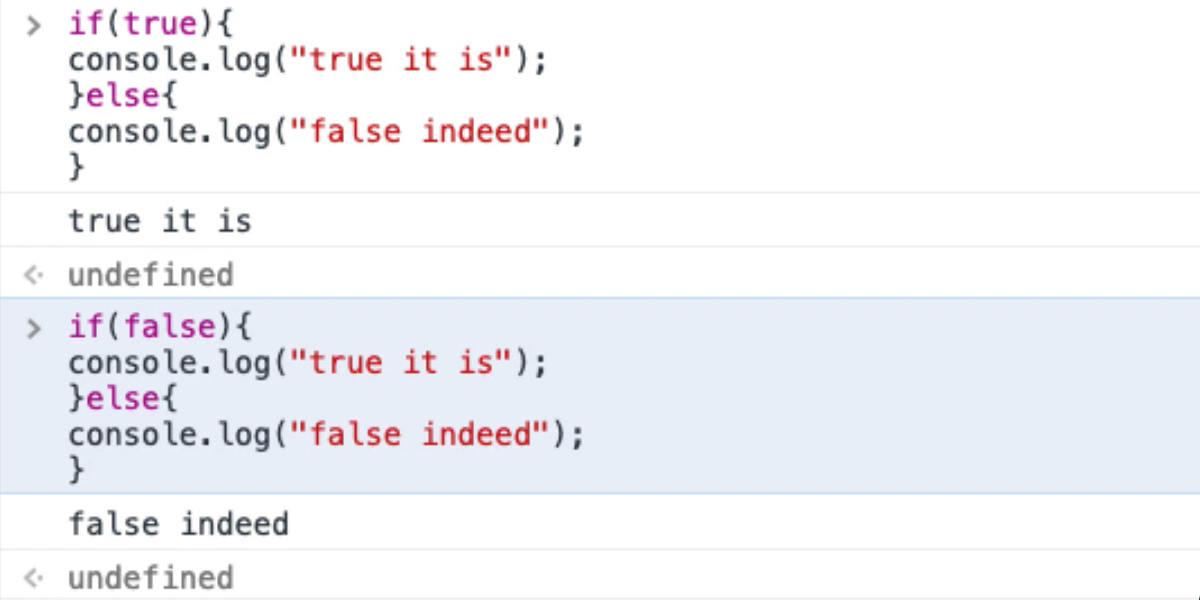So, we've got an `else` statement and I can nest `if` statements in there as well and get really complicated conditional statements, but we don't really need to worry about that for this application.